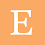## Pages

### Monte Carlo: Roll 2 Dice

Sometime ago I posted about the Sum of Two Dice. In that post, I explained the math behind it and I also performed a Monte Carlo Simulation in R. I would like to go back to this problem to show how my free Monte Carlo Simulation Excel file can be used in this situation.

Assuming a fair die, each die has six different possible output values (1, 2, 3, 4, 5, and 6) and the probability of any of these values should be equal (1/6). The probability function that describes this behavior is the uniform discrete probability function with a lower bound of 1 and an upper bound of 6. The reason that this is a discrete probability is that in between values that are not integers (such as 1.5) are not an option.

Using my Monte Carlo Simulation Excel file to simulate the sum of two dice is very straight forward. First, click "0. Reset"  in the Simulator sheet to make sure you are starting with a fresh and clean sheet. Indicate that the number of inputs is 2 by typing this value in cell F5. Remember, each input will represent one die. Now, click on "1. Define Inputs" and select the Uniform_Discrete probability function for both inputs with 1 as the lower bound (parameter 1) and 6 as the upper bound (parameter 2). Leave the other parameters empty. Your Simulator sheet should look like this:
Now indicate the number of simulations to perform by typing the number of simulations in cell F6. For this example, I will perform 1 million simulations knowing that this large number of simulations will likely take some time to complete so I will have to be patient. It took ~30 mins in a very slow computer on battery. Note that if I would have done 100K simulations it would have only taken about 3 minutes (time is approximately linear with number of simulations). Faster computers will finish faster.

Once the simulation is completed, there are a couple of things to do. First, in the "Y=" column, you will want to type the equation which is basically the sum of die 1 and die 2. This should look like this:

First, let's explore the output of each die. My Monte Carlo Simulation Excel file automatically outputs a histogram of your X1 variable if you have excel 2016 or higher. However, for discrete outcomes, I like making my own using the Excel Function COUNTIF. Doing this I obtained very similar outputs for both X1 and X2. Below I show the output for X1. Note that the number of occurrences for each number is about 1/6 of the total as expected.

In the same way, we can plot the histogram of our Y= X1 + X2. Note that in accordance with my previous post, the number 7 occurred approximately 16.6667% of the time. It is pretty interesting how the sum of two variables that have a uniform discrete probability function results in an output variable with a discrete triangular distribution function.

Now that we have looked into the sum of 2 dice, how about the sum of 3 dice, or 7 dice? Can you use my Monte Carlo Simulation Excel file to do this with more than 2 dice?

#### 1 comment:

1.